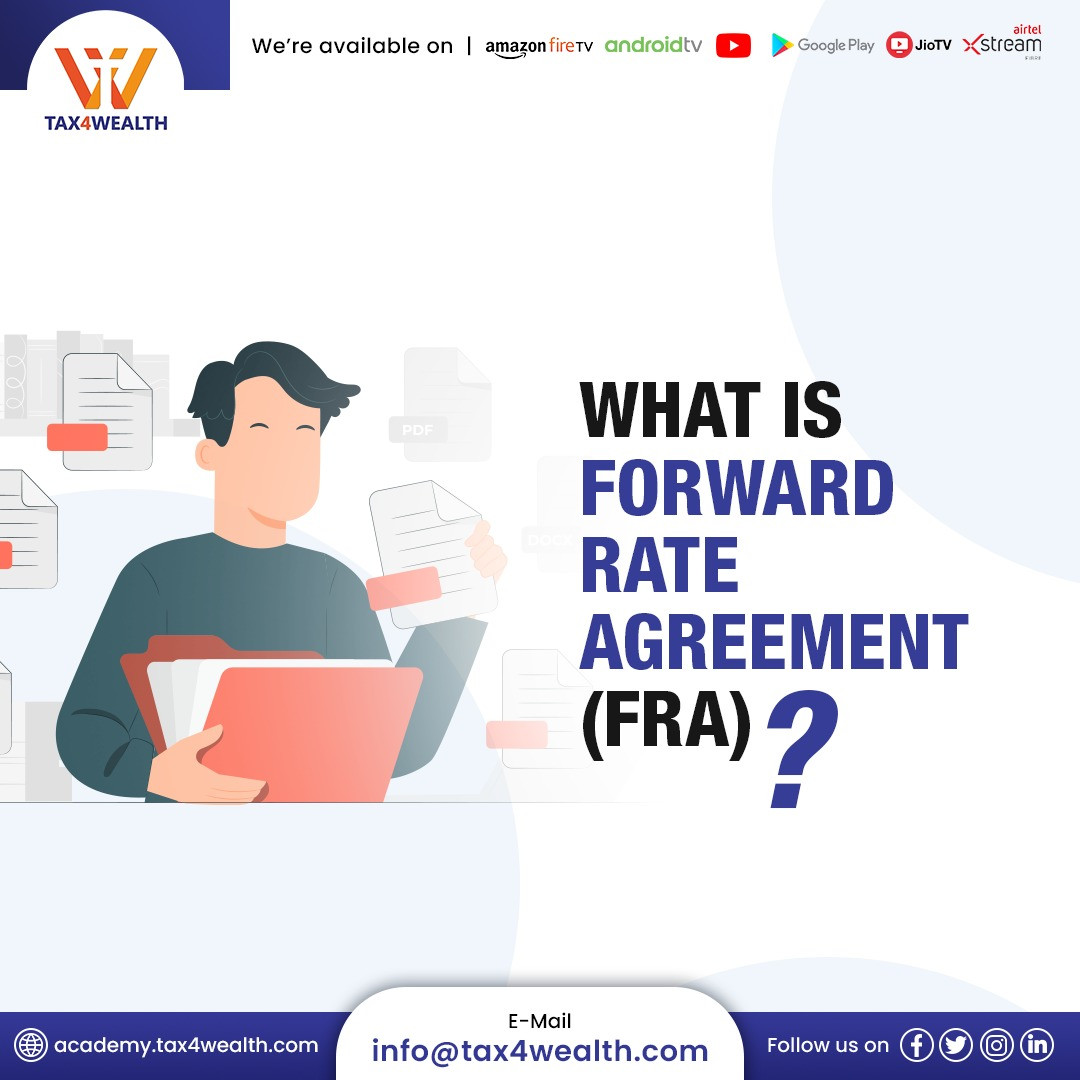# What is Forward Rate Agreement (FRA)?#### What is Forward Rate Agreement (FRA)?

Forward Rate Agreement widely Known as FRA, is an over the counter (OTC) arrangement between the parties that ascertain the interest rate for the contract on a prearranged date in the future.

To rephrase it, a Forward Rate Agreement is an arrangement that is initiated to exchange an interest rate commitment on a speculative amount. The FRA establishes the rates of interest to be imposed and the date of termination and the notional amount along with it.

FRA is a contract between two parties at a predetermined interest rate, where none of the parties gains any profit or bears any loss from this arrangement.

Furthermore, The Forward Rate Agreement is settled with Cash. The Payment is determined on the basis of the net difference between the floating rate (reference rate) in the market and the rate of interest of the contract. This is a cash-based amount determined by the differences between the rate and notional value which is why the notional amount is not exchanged.

## How does the Forward Rate Agreement Work?

The number of parties involved in an FRA is two parties, the buyer and the seller. Under this arrangement, the buyer fixes the Rate of Borrowing at the contract’s formation while the seller is in charge of fixing the lending rate. At the initiation of an Forward Rate Agreement, none of the parties has any profit or loss.

However, in the case where some time has passed the buyer may develop some profit when the interest rate increases than the rate fixed at the time of formation of the agreement, meanwhile the seller benefits if the lending rate drops than the rate fixed at the time of contract formation. In other words, the Forward Rate Agreement creates a situation that is like a Zero-sum game where the gain of one is a loss for the other.

## The Formula of Forward Rate Agreement

FRAP= {(R−FRA)×NP×P] / Y} × {1/ [ 1+R×(Y/P)] }

Where:

FRAP= FRA Payment

FRA= interest rate fixed under the FRA agreement

R= Reference or Floating Rate

NP= Notional Principal or Notional Amount on which interest rate is applied to.

P= Period or the number of days specified under the contract

Y= Number of days in the year specified by the correct day count for the contract## Related News#### Equity Swap: Its Usage, Valuation and Accounting Treatment#### What is Forward Rate Agreement (FRA)?